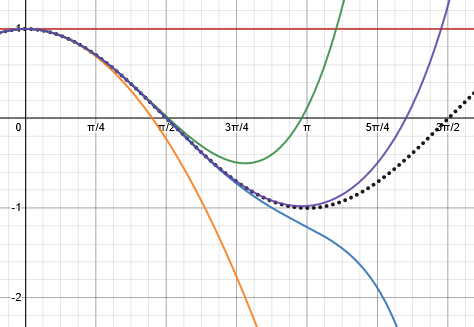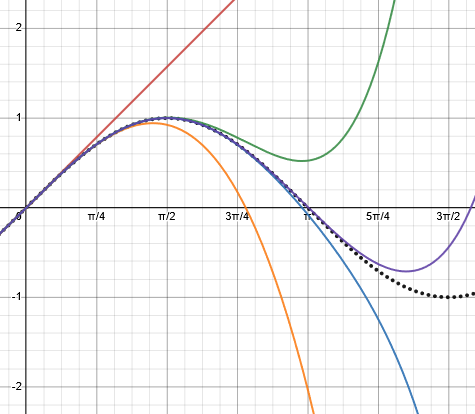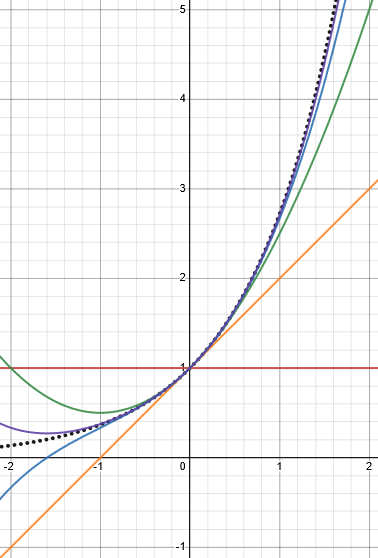# Euler’s Formula: Complex Numbers as Exponents

Last week we explored how the polar form of complex numbers gives multiplication a simple geometric meaning. Here we’ll go one more step, and express polar form exponentially, which makes DeMoivre’s theorem trivial, and gives us a simple notation to replace “cis”.

The fact that multiplying complex numbers results in adding their angles, as we saw last week, provides a hint to what’s coming: Since that is exactly what exponents do (multiplying powers of the same base adds their exponents, as in $$a^m\cdot a^n=a^{m+n}$$, maybe complex numbers are exponential functions of the angle? In fact, that turns out to be true. The amazing formula, discovered by Euler, tells us that $$e^{ix}=\cos(x)+i\sin(x)$$ And this means that $$e^{i\theta}$$ is another way to write what we wrote last time as $$\text{cis}(\theta)$$, with even fewer letters.

In particular, if we let $$x=\pi$$, we get $$e^{i\pi}=\cos(\pi)+i\sin(\pi)=-1.$$

In the form $$e^{i\pi}+1=0$$ it is often pointed out that this equation involves the five most important numbers in mathematics, 0, 1, e, i, $$\pi$$.

But why should we believe this formula? And how can we believe it? That is today’s subject.

## Plausibility checks

Proof of e^(ix) = cos(x) + isin(x)

In the equation e^(iPi) + 1 = 0, the proof is to evaluate

e^(ix) = cos(x) + i sin(x) for x = Pi.

I would like to see a rigorous proof of the above equation.

Doctor Mitteldorf answered by providing several ways to think about it, including a proof:

Dear Walter,

This is called the Euler equation, and it's not something you can prove rigorously.  It's a definition, and I'd like to convince you that it's the only sensible definition, of how to compute imaginary exponentials.

We’ll be seeing two or three proofs; so why does he say it can’t be proved? Because until we write this equation, its left-hand side has no meaning! It’s a lot like what we’ve said about, say, multiplying negative numbers: We need to define it before we can prove anything about it. But the definition we give is the only definition that would allow everything else we know to continue working. So it really is a proof … not that this is abstractly true, but that we have to define it this way.

I can think of three approaches to verifying the Euler equation, but unfortunately one of them is all calculus, one uses calculus explicitly, and only the third is free of calculus.  I'm just guessing from your age that you may not have studied calculus yet.

You can verify that the Euler equation makes a sensible definition by expanding the two sides as Taylor series in x.  You can also differentiate both sides and see that the answer is self-consistent.  Thirdly, you can use the formula for cos(2x) and sin(2x) to show that the right side has the property you expect from an exponential, so that e^(i(2x)) = (e^(ix))^2.

### Compare to what we know

This third approach is just a plausibility check; it amounts to the idea I mentioned at the top, that the way multiplication works looks like an exponential.

So start with choice 3.  You have the formulas

cos(2x) = cos^2(x) - sin^2(x) and
sin(2x) = 2 sin(x) cos(x)

You'd also want to demand that e^(i(2x)) = (e^(ix))^2.  That means that your new definition of e^(ix) is behaving like an exponential.  See if you can put these together to show that

e^(i(2x)) = cos(2x) + i sin(2x).

So if $$e^{ix}=\cos(x)+i\sin(x)$$ for all x, then replacing x with 2x, it must be true that $$\left(\cos(x)+i\sin(x)\right)^2=\left(e^{ix}\right)^2=e^{i\cdot2x}=\cos(2x)+i\sin(2x)$$. If so, then we have a little piece of evidence that the formula makes sense. So what do we get when we square the left-hand side? $$\left(\cos(x)+i\sin(x)\right)^2=\cos^2(x)+2i\sin(x)\cos(x)+i^2\sin^2(x)\\=(\cos^2(x)-\sin^2(x))+(2\sin(x)\cos(x))i\\=\cos(2x)+i\sin(2x)$$

What he’s saying here can be enlarged: This definition is compatible with DeMoivre’s theorem, which says that any power of a complex number multiplies its angle by the same number. We’ll see that again later.

And, similarly, we could show that this definition is compatible with the way products of complex numbers work, adding the angles: $$e^{ix}e^{iy}=(\cos(x)+i\sin(x))(\cos(y)+i\sin(y))\\=\cos(x)\cos(y)+i\cos(x)\sin(y)+i\sin(x)\cos(y)-\sin(x)\sin(y)\\=(\cos(x)\cos(y)-\sin(x)\sin(y))+i(\cos(x)\sin(y)+\sin(x)\cos(y))\\=\cos(x+y)+i\sin(x+y)=e^{i(x+y)}$$ as we would expect.

### Taylor series

The Taylor expansion is something you can appreciate without calculus, although its roots are in calculus.  It's a series expression for a function. You may have run across the following infinite series representations of cos and sin and e^x.  In fact, this is the most straightforward way to compute the value of sin(x) or e^x for any given x.

cos(x) = 1 - x^2/2!   + x^4/4!   - x^6/6! + ...
sin(x) = x - x^3/3!  + x^5/5!  - x^7/7! + ...
e^x = 1 + x + x^2/2! + x^3/3! + x^4/4! + ...

The ! in these equations means factorial.  In other words, 4! = 4*3*2*1.

See if you can use these infinite series expressions to verify the Euler equation.

Students often ask how calculators work out sines and cosines, and these series (though not what is actually used by calculators) are part of the answer. If you have never seen them, you may be convinced (remember, we aren’t doing proofs yet, just checking plausibility) by these graphs:

Here are the first one, two, three, four, five terms of the cosine series:Similarly, here are the first one, two, three, four, five terms of the sine series:And here they are for the $$e^x$$ series:In each case you can see that just the first few terms approximate the curve closely for x close to zero, and the more terms you add, the closer you come for any x.

Now, if the series for the exponential function works for complex exponents, what will we get?

$$e^{ix}=1+(ix)+\frac{(ix)^2}{2!}+\frac{(ix)^3}{3!}+\frac{(ix)^4}{4!}+\dots\\=1+ix-\frac{x^2}{2!}-i\frac{x^3}{3!}+\frac{x^4}{4!}+\dots\\=(1-\frac{x^2}{2!}+\frac{x^4}{4!}+\dots)+i(x-\frac{x^3}{3!}+\dots)\\=\cos(x)+i\sin(x)$$

So, if we can expect the exponential series to “work” for an imaginary argument, then the formula is correct.

After you complete these two projects, I'm hoping you'll find the Euler equation very plausible.  If you still want more proof, write back again...

### Differentiation: another check for compatibility

Doctor Mitteldorf had given a very similar answer to another question a couple months earlier:

Euler Equation

There he included a mention of his purely calculus approach:

3. If you know a little calculus, you can try differentiating the Euler equation to see that you get a consistent answer.

There are branches of physics where people are constantly using the Euler equation to go back and forth between the trigonometric and the exponential version of a formula, because first one version then the other turns out to be easier to work with.  To them, the Euler equation has become second nature.

This will be another confirmation, rather than a derivation/proof; we’ll see the latter soon. Let’s do what he said:

Differentiating both sides of $$e^{ix}=\cos(x)+i\sin(x)$$ (again, assuming that all the rules we are used to still apply) we get $$ie^{ix}=-\sin(x)+i\cos(x).$$ Multiplying through by $$-i$$ (which is equivalent to dividing by $$i$$), we get $$e^{ix}=i\sin(x)+\cos(x),$$ which is what we started with, which means differentiation still works. The formula is consistent.

Here’s another question from 1997:

Euler Equation

I have seen the famous Euler equation e^i*pi-1 = 0 and know something about its derivation. Specifically, it follows from the infinite series that define sin, cos and e^x. My question is: does the equation still work if we decide to work in degrees (i.e. is e^i*90-1=0 true)?

I ask because the mysterious appearance of e, i, and pi in one equation isn't so strange if it is partly definitional. I believe the question boils down to this: do the infinite series definitions of sin and cos

(cos(x) = 1 - x^2/2! + x^4/4! - x^6/6! + ..., and
sin(x) = x - x^3/3! + x^5/5! - x^7/7! + ...)

work if x is expressed in degrees, or are they only valid when x is a radian expression?

Oliver has seen the derivation from the Taylor series, and is partly confusing the general formula and the special case. But does it depend on using radians?

Doctor Anthony answered, first correcting a small error:

The Euler equation is  e^(i.pi)+1 = 0

The series you quote for cos(x) and sin(x) require x to be in radians.  Radian measure is non-dimensional, being the ratio of two lengths, arc length divided by radius, just as sin and cos are non-dimensional, again being ratios of lengths.  It is necessary, of course, that the two sides of the equation have the same dimensions - in this case both are non-dimensional.

Degrees are not mathematical in any real sense.  They are arbitrary conventions and, historically, mathematicians could have agreed to have 500 'degrees' in a circle or any number you care to think of.

In general, trig functions as used in calculus assume the argument is in radians, which are defined by inherent properties of the unit circle (whereas degrees are defined for human convenience); and that extends to the exponential function for complex exponents. So in reality, $$e^{90i}=\cos(90rad)+i\sin(90rad)\approx-0.448+0.894i,$$ not $$\cos(90^\circ)+i\sin(90^\circ)=0+1i=i,$$ or, as Oliver probably meant to say, $$\cos(180^\circ)+i\sin(180^\circ)=-1+0i=-1.$$ Rather, $$e^{i\pi}=\cos(\pi rad)+i\sin(\pi rad)=-1+0i=-1.$$

Oliver wrote back, wondering about what is “arbitrary” and what is not:

Thank you very much for your reply. It was quite helpful. I am still a little confused.  I have heard in a number of places that the Euler function is in some way arbitrary. From the information in your email, it seems to me that one could fairly easily prove the truth of the equation. To me that indicates that the Euler function tells us something "real" and not constructed. Am I missing something?

Thanks again for the help you have already given.

Interestingly, Oliver was aware of a derivation, but also believed it to be unprovable. These concepts are hard to fully appreciate before you have a lot of experience with upper level mathematics; the idea he’d heard may come from the fact we saw at the beginning, that it is not technically a proof, but a (justifiable) definition.

### Proof by differentiation

Doctor Anthony replied with a calculus proof that amounts to reversing the plausibility check we did with derivatives:

The Euler equation is indeed something real. The derivation is not too difficult if you are familiar with the basics of complex numbers and exponential functions.

dz/dx = -sin(x) + i.cos(x)

= i(cos(x) + i.sin(x))    (since i^2 = -1)

= i.z

So   dz/z = i.dx          now integrate both sides

ln(z) = i.x + const.  from (1) when x= 0, z= 1
so const. = 0
ln(z) = i.x

z = e^(i.x)    but z = cos(x) + i.sin(x) so

cos(x) + i.sin(x) = e^(i.x) .........(2)

Put x = pi in (2) and we get:

-1 + 0 = e^(i.pi)

and so e^(i.pi) + 1 = 0

As you can see there is nothing contrived about this equation.

Looking closely, we see that he is assuming that derivatives and integrals work as expected for complex numbers; a fuller foundation would be needed in order to make this more than just a proof that Euler’s formula is the definition that allows calculus to continue to work.

Also, if we keep going far enough to examine complex logarithms, we’ll run across the fact that the log of a complex number is multi-valued, which should be treated carefully. But such issues don’t invalidate the conclusion.

This proof was copied into our FAQ on this subject. The following question from 1999 asks about it:

Euler's Equation: First Step

http://mathforum.org/dr.math/faq/faq.euler.equation.html

I have a question on the very first step: any complex number, z, equals cos(x)+isin(x). Why is this? Is this a definitional thing, that any complex number can be expressed in this fashion?

Max is referring to the first line of the proof, “Start with z = cos(x) + i.sin(x)”.

Dear Max,

Of course it's not true that any complex number can be expressed as cos(t)+i*sin(t). For example, the number 2 is a complex number that cannot, because there's no x for which cos(t) = 2. Any complex number with UNIT MODULUS can be expressed as cos(t)+i*sin(t), i.e., any complex number that obeys |z|^2 = 1.

The statement in the proof doesn’t say, “start with any z, and you can write it as $$\cos(x)+i\sin(x)$$, but rather, “let $$z=\cos(x)+i\sin(x)$$, so that the right-hand side is what we are working with (a complex number with modulus 1), and we are calling it z.”

What if you did want to work with an arbitrary z?

So starting with any z at all, you can first write it as R times z', where z' has unit modulus, and R is the modulus |z|. Then it is true that z' can be expressed as cos(t)+i*sin(t). Just let x be the angle whose tangent is the quotient of the imaginary part of z to the real part of z.  In other words, take the imaginary part of z and the real part, and divide them. Think in terms of the complex plane where the y axis represents the imaginary part and the x axis the real part. The angle t is formed when you connect the point x+iy to the origin. The length of the connection is R=|z|. Basic trig definitions give the imaginary part as R sin(t) and the real part as R cos(t).

In other words, we can put any complex number into polar form, and $$z’=\frac{z}{|z|}$$ will have the form used in the proof. But the fact we are proving is only about $$\cos(x)+i\sin(x)$$, which has modulus 1.

## DeMoivre’s theorem, reprise

DeMoivre’s theorem, which we closed with last week, is so closely related to Euler’s formula that I (and others) sometimes forget which is which if we’ve been away from it for a while. Let’s close with a question from 1998 about it:

DeMoivre's Formula

I have forgotten about DeMoivre's formula. Thanks in advance for the reminder.

Hi Greg,

I believe the formula you're looking for is DeMoivre's formula, which is the following:

(cos(theta) + i*sin(theta))^n = cos(n*theta) + i*sin(n*theta)

This formula is useful when you have a complex number and want to raise it to some power without doing a lot of work.

If all you want is the formula, you can ignore the rest of this message. However, if you want a little more insight into what is going on, read on.

We proved this last time inductively, assuming that n is a positive integer. But now that we know Euler’s formula, we can do more:

Recall that any complex number can be written in the form r*e^(i*theta). If you plot a complex number in the complex plane (where the x-axis is the real axis and y-axis is the imaginary axis), then "r" will be the distance from the point to the origin and theta will be the angle a line from the origin to the point makes with the x-axis. A little trig shows that a complex number written as r*e^(i*theta) can also be written as r*cos(theta)+r*i*sin(theta).

It’s more than trig, but this is what we’ve “proved” above.

Knowing this little fact gives us the ability to switch back and forth between ways of writing complex numbers, depending on what we want to do with them. If we want to add complex numbers, then the form a + b*i is easiest, whereas if we want to multiply them together, it is easier to use the form r*e^(i*theta).

In other words, the rectangular form makes addition (and subtraction) easy; the polar form, however you choose to write it, make addition (and division) easy.

Essentially what you are doing is taking a complex number of the form (a + b*i), converting it to the form r*e^(i*theta), raising it to a power in that form, then converting back to the first form.  Observe:

(r*cos(theta) + r*i*sin(theta))^n
= (r*(cos(theta) + i*sin(theta))^n
= (r^n) * (cos(theta) + i*sin(theta))^n
= (r^n) * (e^(i*theta))^n
= (r^n) * (e^(n*i*theta))
= (r^n) * (cos(n*theta) + i*sin(n*theta))

So DeMoivre is just a simple application of Euler. This higher perspective makes what was hard become easy. And we didn’t assume anything about n!

(Well, again, there is something hidden here. As we’ll see when we look at complex roots, everything becomes multi-valued. This is true even for square roots of positive real numbers, though there we are able to work around it by choosing a principal root. That can’t be done for complex numbers in a way that eliminates the ambiguity. But this fact is still as true as it can be!)

Of course knowing DeMoivre's formula allows us to go straight from

(r*(cos(theta) + i*sin(theta))^n

to

(r^n) * (cos(n*theta) + i*sin(n*theta)).

Greg replied,

Hi Dr. Math,

That was way more than I was looking for. I found it quite interesting and am glad to know it.

Greg